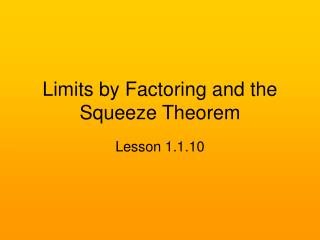DownloadDownload PresentationLimits by Factoring and the Squeeze Theorem

# Limits by Factoring and the Squeeze Theorem

Télécharger la présentation## Limits by Factoring and the Squeeze Theorem

- - - - - - - - - - - - - - - - - - - - - - - - - - - E N D - - - - - - - - - - - - - - - - - - - - - - - - - - -
##### Presentation Transcript

1. Limits by Factoring and the Squeeze Theorem Lesson 1.1.10

2. Learning Objectives • Given a rational function, evaluate the limit as xc by factoring the numerator and/or denominator. • Given a function, evaluate the limit as xc using the Squeeze Theorem.

3. Review of Factoring • For this lesson, you need to know all types of factoring from Algebra II/Pre-calc • This includes: • GCF factoring • Quadratic trinomial factoring • Difference of squares • Sum/difference of cubes • Synthetic division

4. GCF Factoring • x3 – 5x • 2x + 16

5. Quadratic Trinomial Factoring • x2 + 5x + 6 • x2 – 5x + 4 • x2 + 3x – 4 • x2 – 7x – 30

6. Difference of Squares a2 – b2 factors to (a + b)(a – b) • x2 – 4 • x2 – 9 • 9x2 – 25 • 4x2 – 49

7. a3 + b3 factors to (a + b)(a2 – ab + b2) x3 + 27 8x3 + 125 a3 – b3 factors to (a – b)(a2 + ab + b2) x3 – 125 27x3 – 8 Sum/Difference of Cubes

8. Synthetic Division • This will be useful for polynomials of degree 3 or higher x3 + 4x2 + x – 6 • Typically, you would use p/q to figure out which roots to try. • The c value of the limit, however, will likely be one of the roots. Try that first.

9. Factor x3 + 4x2 + x – 6. c value is -2

10. We can evaluate a limit by factoring the numerator and/or denominator and “canceling out” like factors. After canceling out, we just plug in the c value. Evaluating Limits by Factoring

11. Example 1 • Evaluate the following limit.

12. Example 2 • Evaluate:

13. Example 3

14. Example 4

15. Example 5

16. Factoring Wrap-Up • Thought question: Do you believe that the two functions on the right are equal? Why or why not?

17. Squeeze Theorem • Suppose the function f(x) is in between two other functions, g(x) and h(x) • Not only that, but as the limit as xc for g(x) and h(x) is equal. • As a result, the limit of f(x) as xc must be the same as for g(x) and h(x).

18. More Formally… Sometimes, this Squeeze Theorem is known as the Sandwich Theorem.

19. Example 6 Use the Squeeze Theorem to find given

20. Applying the Squeeze Theorem • Suppose that you were told to evaluate the limit below. • Calculating this limit on its own is very difficult. • This function, however, is “squeezed” in between two functions with the same limit. This limit is easy to find.

21. Remember:the cosine of any angle must be in between -1 and 1. • Thus, when you multiply any number by a cosine, its magnitude becomes smaller. • Therefore, we can make the following argument:

22. Visually

23. Therefore… • Let’s instead take the limits of -|x| and |x| as x0 • You can just plug in 0 for x. • You will get a limit of 0 for both functions. • Because x*cos(1/x) is in between these two functions, and both have limits of 0, x*cos(1/x) must also have a limit of 0 as x0.

24. Wrap-Up • You can use factoring to determine fraction limits algebraically. • You can find the limit of a function in between two functions using the Squeeze Theorem.

25. Homework • Textbook 1a-d, 2